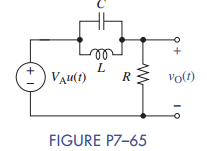Create an Account

Home / Questions / The circuit in Figure P7–65 is in the zero state when the step function input is applied. ...

The circuit in Figure P7–65 is in the zero state when the step function input is applied. (a) If V...

The circuit in Figure P7–65 is in the zero state when the step function input is applied.

(a) If VA = 15 V, R = 1.5 kΩ, L = 250 mH, and C = 0.25 μF, derive an expression for the voltage vO(t) for t ≥ 0.

(b) Validate your solution by plotting it using MATLAB and comparing it to a Multisim simulation of the same circuit.Jul 28 2020 View more View LessSubscribe To Get Solution